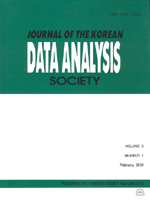상세검색
최근 검색어 전체 삭제
다국어입력
즐겨찾기0KCI등재 학술저널

# Variable Selection in Multivariate Data

• 등재여부 : KCI등재
• 2011.12
• 2795 - 2801 (7 pages)

A problem of selecting a subset of the original variables based on the likelihood is considered. An appropriate perturbation scheme is attached to the variables and the likelihood displacement is computed that forms a curve in the Euclidean plane. The direction vector associated with the maximum curvature of this curve provides information about a variable that greatly change the likelihood displacement. Variables associated with large absolute components of the direction vector would be included in the model. A numerical example is given for illustration. The simulated data set shows that the variable selection procedure works well. This procedure of choosing variables would be useful for analyzing multivariate data.

1. Introduction

2. A procedure for selecting variables

3. Detailed derivations

4. A numerical example

References